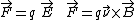# Aharonov-Bohm effect

(redirected from AB effect)

## Aharonov-Bohm effect

The predicted effect of an electromagnetic vector or scalar potential in electronic interference phenomena, in the absence of electric or magnetic fields on the electrons.

The fundamental equations of motion for a charged object are usually expressed in terms of the magnetic field and the electric field. The force on a charged particle can be conveniently written as in the equations below, where q is theparticle's charge, is its velocity, and the symbol × represents the vector product. Associated with is a scalar potential V defined at any point as the work W necessary to move a charge from minus infinity to that point, V = W/q. Generally, only the difference in potentials between two points matters in classical physics, and this potential difference can be used in computing the electric field. Similarly, associated with is a vector potential, a convenient mathematical aid for calculating the magnetic field. See Electric field, Potentials

In quantum mechanics, however, the basic equations that describe the motion of all objects contain and V directly, and they cannot be simply eliminated. Nonetheless, it was initially believed that these potentials had no independent significance. In 1959, Y. Aharonov and D. Bohm discovered that both the scalar and vector potentials should play a major role in quantum mechanics. They proposed two electron interference experiments in which some of the electron properties would be sensitive to changes of or V, even when there were no electric or magnetic fields present on the charged particles. The absence of and means that classically there are no forces acting on the particles, but quantum-mechanically it is still possible to change the properties of the electron. These counterintuitive predictions are known as the Aharonov-Bohm effect.

Surprisingly, the Aharonov-Bohm effect plays an important role in understanding the properties of electrical circuits whose wires or transistors are smaller than a few micrometers. The electrical resistance in a wire loop oscillates periodically as the magnetic flux threading the loop is increased, with a period of h/e (where h is Planck's constant and e is the charge of the electron), the normal-metal flux quantum. In single wires, the electrical resistance fluctuates randomly as a function of magnetic flux. Both these observations, which were made possible by advances in the technology for fabricating small samples, reflect an Aharonov-Bohm effect. They have opened up a new field of condensed-matter physics because they are a signature that the electrical properties are dominated by quantum-mechanical behavior of the electrons, and that the rules of the classical physics are no longer operative. See Quantum mechanics

## Aharonov-Bohm effect

[‚ä·hä′rō‚nȯf ′bām i‚fekt]
(quantum mechanics)
An effect manifested when a beam of electrons is split into two beams that travel in opposite directions around a region containing magnetic flux and are then recombined, whereby the intensity of the resulting beam oscillates periodically as the enclosed magnetic field is changed.
References in periodicals archive ?
0]A(x) associated with it is present, then the wave function undergoes a change of the phase factor by the AB effect.
or -]]([zeta]) describes the AB effect term along the trajectory from [d.
0] describes the AB effect arising from the trajectories from [d.
The AB effect refers to the finding that T2 is identified less well when the temporal interval (i.
s results clearly implied that the reduced AB effect for arousing taboo words was due to a more efficient processing of taboo stimuli than neutral stimuli.
1997), we found no AB effect for participants' own names, whereas we did find a strong AB effect for control names.
The AB effect refers to the impairment for identification or detection of the second target (T2) when presented briefly (within 200-600 ms) after the first target (T1) (Raymond, Shapiro, & Arnell, 1992).
Basically, the AB effect can be viewed as an attentional switch cost reflecting the difficulty to switch attention from T1 to T2 when it appears briefly after T1.
We reasoned that if temporal and spatial switches of attention on T2 are sufficiently strong at the shortest lags, then the AB effect should still be observed even when no distractor follows T2.
3) There is no other way of understanding the AB effect except by taking wave packets as physically real, as will be seen in the next section.
Before we discuss the AB effect, let us see what becomes of (R) from our discussion above.
Very much like the EPR thought experiment, the AB effect was first predicted by Aharonov and Bohm in 1959 as the result of a thought experiment (Aharonov and Bohm ) and later experimentally confirmed (cf.
Site: Follow: Share:
Open / Close